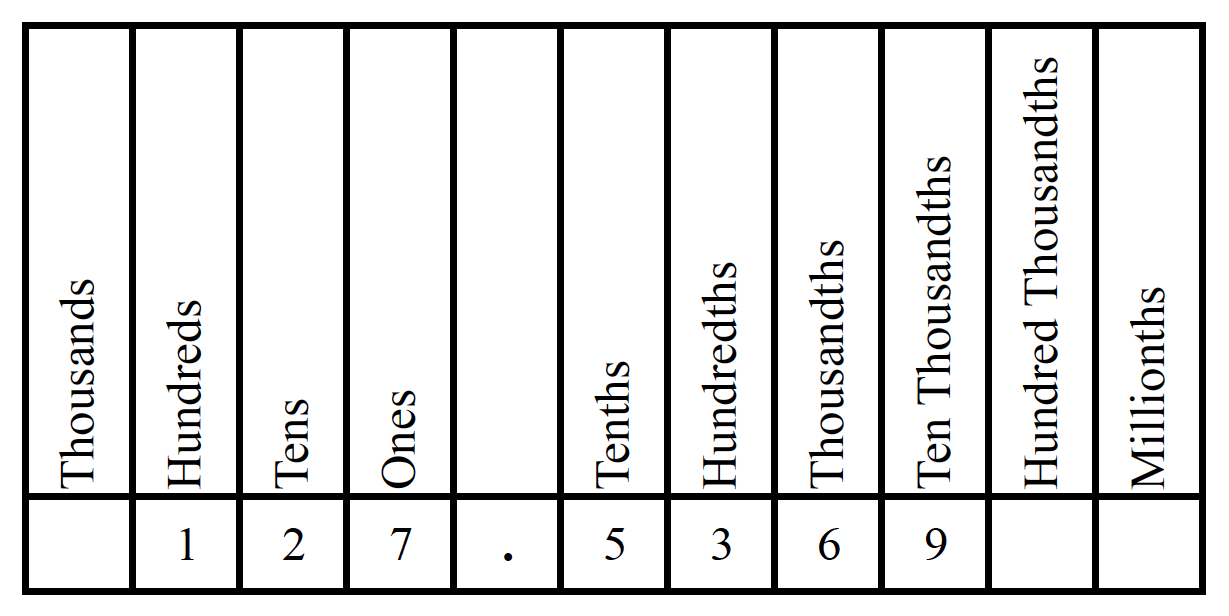### Home > CC1 > Chapter 1 > Lesson 1.1.3 > Problem1-20

1-20.

The value of a decimal becomes clearer when the place value is spoken or written as the number it names. For example, $0.1$ makes more sense if it is read as “one tenth” rather than “zero point one.” Homework Help ✎

1. Write the following numbers in words so that the place value can be identified.

$0.4$

$1.3$

$0.56$

$2.008$

• Make sure to pay attention to the last digit place of the decimal value. Use the chart below for guidance.

•• Look to see if there is a number to the left of the decimal; if so write it out. If there is no number to the left of the decimal, skip to step 3.

• Include 'and' for the decimal point if there is a number to the left of the decimal.

• Write out the number to the right of the decimal up to the last digit. Write the place value of the last digit.

$0.64 = \text{sixty-four hundredths}$

With these steps, try writing each number in words.

1. Now reverse your thinking. Write the decimals that go with the following words.

thirty-five hundredths

three and two tenths

six hundredths

• Refer to part (a) for help with decimal places.

• Consider the significance of the place value identified by the last word.

$\text{thirty-fivehundredths}=0.35$